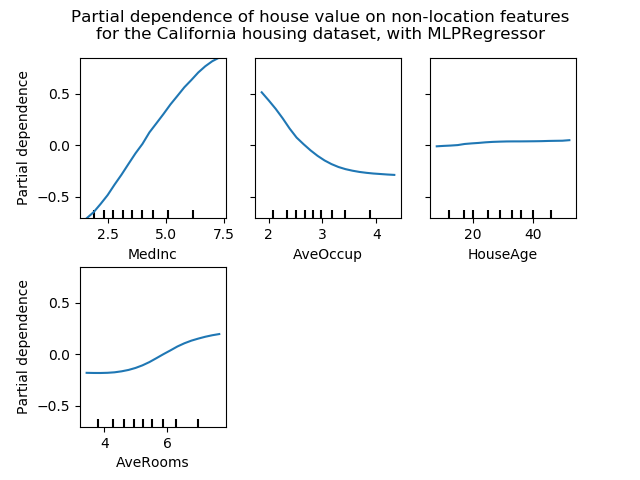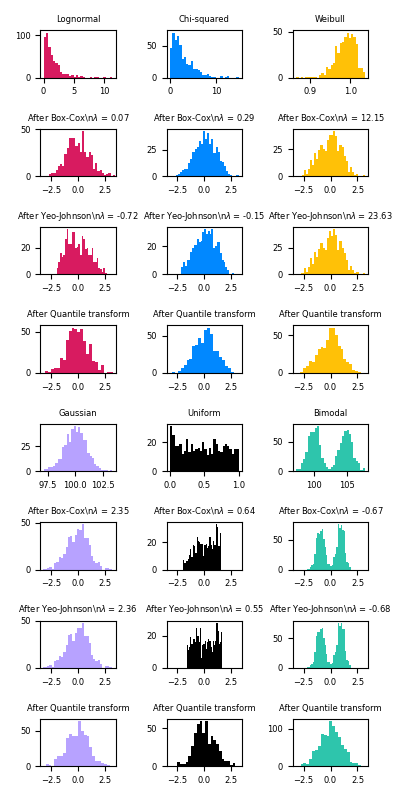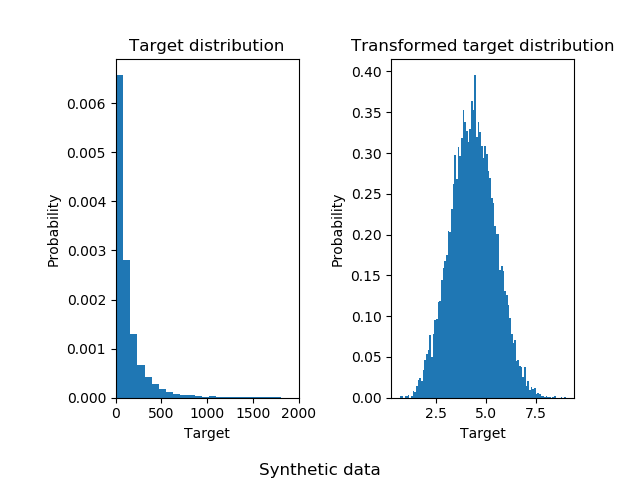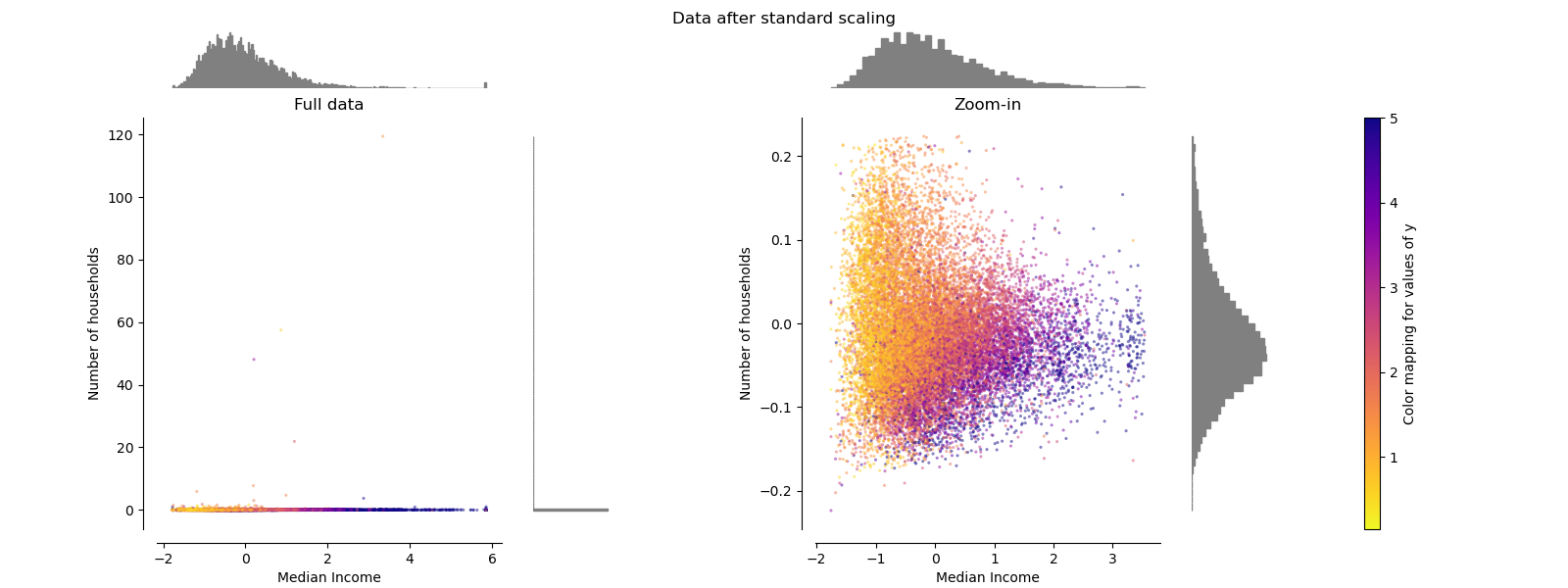# sklearn.preprocessing.QuantileTransformer¶

class sklearn.preprocessing.QuantileTransformer(*, n_quantiles=1000, output_distribution='uniform', ignore_implicit_zeros=False, subsample=100000, random_state=None, copy=True)

[源码]

n_quantiles int, optional (default=1000 or n_samples)

output_distribution str, optional (default=’uniform’)

ignore_implicit_zeros bool, optional (default=False)

subsample int, optional (default=1e5)

random_state int, RandomState instance or None, optional (default=None)

copy boolean, optional, (default=True)

n_quantiles_ integer

quantiles_ ndarray, shape (n_quantiles, n_features)

references_ ndarray, shape(n_quantiles, )

quantile_transform

PowerTransformer

StandardScaler

RobustScaler

NaN被视为缺失值：忽略适合度，并保持变换值。

>>> import numpy as np>>> from sklearn.preprocessing import QuantileTransformer>>> rng = np.random.RandomState(0)>>> X = np.sort(rng.normal(loc=0.5, scale=0.25, size=(25, 1)), axis=0)>>> qt = QuantileTransformer(n_quantiles=10, random_state=0)>>> qt.fit_transform(X)array([...])

fit(X[, y]) 计算用于转换的分位数。
fit_transform(X[, y]) 拟合数据，然后对其进行转换。
get_params([deep]) 获取此估计量的参数。
inverse_transform(X) 反投影到原始空间。
set_params(**params) 设置此估算器的参数。
transform(X) 数据的按特征进行转换。
__init__(*, n_quantiles=1000, output_distribution='uniform', ignore_implicit_zeros=False, subsample=100000, random_state=None, copy=True)

[源码]

fit(X, y=None)

X ndarray or sparse matrix, shape (n_samples, n_features)

self object
fit_transform(X, y=None, **fit_params)

[源码]

X {array-like, sparse matrix, dataframe} of shape (n_samples, n_features)
y ndarray of shape (n_samples,), default=None

**fit_params dict

X_new ndarray array of shape (n_samples, n_features_new)

get_params(deep=True)

[源码]

deep bool, default=True

params mapping of string to any

inverse_transform(X)

[源码]

X ndarray or sparse matrix, shape (n_samples, n_features)

Xt ndarray or sparse matrix, shape (n_samples, n_features)

set_params(**params)

[源码]

**params dict

self object

transform(X)

[源码]

X ndarray or sparse matrix, shape (n_samples, n_features)

Xt ndarray or sparse matrix, shape (n_samples, n_features)

## sklearn.preprocessing.QuantileTransformer使用示例¶